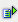The function ceil (num) returns the next higher whole-numbered value of the value (num).

Syntax: =ceil(num)Example =ceil(2.2) is 3 =ceil(-2,2) is -3